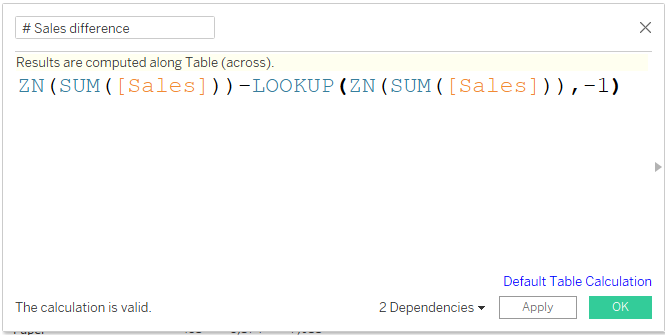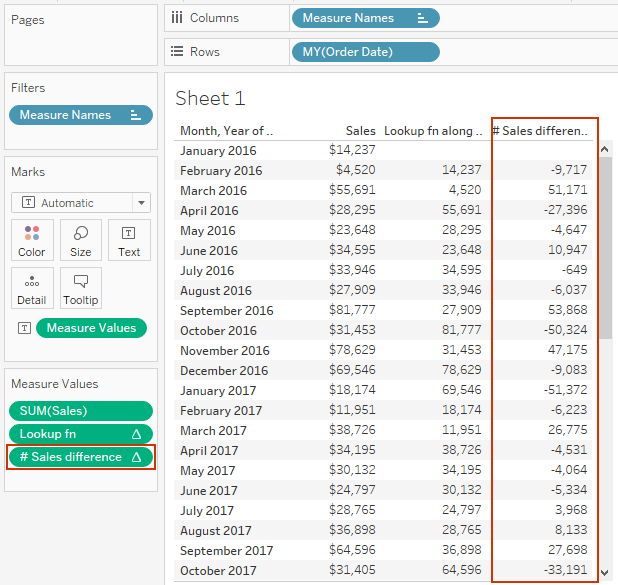top of page
Search
• Bernard K

# Tableau LOOKUP() Function

Updated: Nov 8, 2022This function returns the value of the expression in a target row, specified as a relative offset from the current row. You can use FIRST()+1 and LAST()-1 as part of your offset definition for a target relative to the first/last rows in the partition.

The syntax: LOOKUP(Expression, [Offset])

LOOKUP() function is one of the most useful table calculations in Tableau. In this article, I will demonstrate to you how you can use this function to compute difference and percent difference, respectively.

### Computing difference

Using Superstores data set, I will use this function to compute Sales difference from the previous month for different months of the year. But before that, let’s create the table layout using Month Year of Order Date and Sales as shown below.LOOKUP(SUM(Sales), -1)

Using the above LOOKUP() function, Tableau returns a table with the previous Sales value shifted and aligned with the current value.

Let’s add this new calculation to the view.Therefore, using this function one can compute Sales difference by subtracting the previous value from the current value as shown below;Adding this computation to the view results.(Which is the Sales difference from the previous month’s Sales)

The same calculation can be used to compute Sales difference from the previous year for different product Sub-Category’s, as shown in this table.### Computing percent difference

Extending the above calculation by diving it with the previous value as shown below returns the percent difference.Applying this calculation on the tables, we’ve.If you like the work we do and would like to work with us, drop us an email on our Contacts page and we’ll reach out!